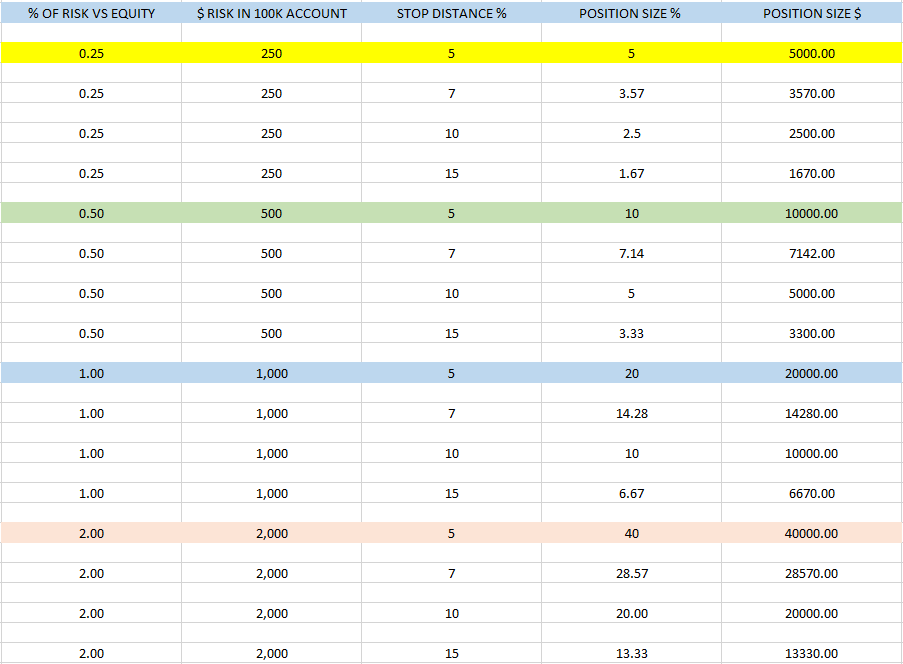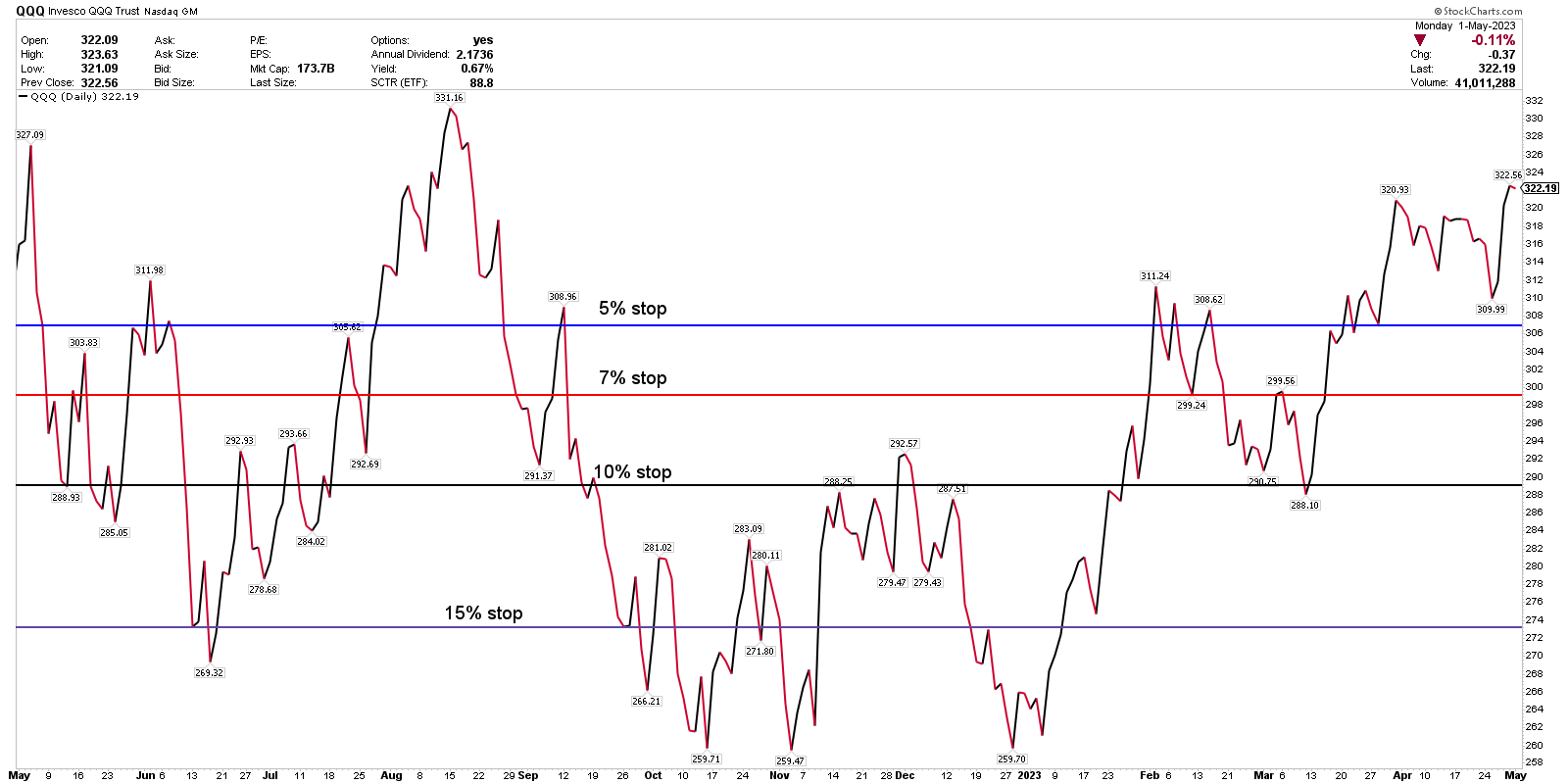# Understanding Position Sizing and Risk Management

Position Sizing and Risk Management

(For purposes of this educational post, a hypothetical \$100,000 account value will be assumed, for ease of calculation).

1. Risk management is one of the most important factors to a trader or investors longevity.
– Always focus on risk management and position management, especially when considering new positions.

2. Total open risk.
– Traders/investors should first determine how much total open risk they want to carry at any one time.
– If ALL positions get stopped out and/or closed, what is the total loss vs portfolio equity, in percentage terms?

3. A key note.
– We will define risk here by the amount that can be lost in the position if it is stopped out, not the total \$ invested.
– Example: If a trader puts \$10,000 into a position with a 5% stop loss, the ‘risk’ here is not \$10,000, it is the 5% potential loss on the \$10,000 investment, or \$500.
– In a \$100,000 account, \$500 risk = 0.50%, or 1/2 of 1%, or 50-basis points. They are all the same.
– Standard ‘total open risk’ levels for many professionals often falls into the 5-10% range, at any one-time.
– This means if all of their positions were stopped out, or closed out, their loss vs account equity would be 5-10%.
– For many professionals, market volatility often dictates how much open risk they will hold.
– In low-volatility, uptrend markets, open risk is often much higher as a target level, than it is in choppy, volatile, news-driven markets.
– For this illustration, we will assume the trader/investor wants to have 5% of ‘total open risk’.

4. Once target ‘open risk’ is determined (5% in our example) – New position risk needs to be calculated before entering a position.

5. New risk also needs to be added to current open risk, to determine ‘total open risk’ or ‘total exposure’.
– The new position risk added needs to be added to current open risk.

6. Position risk.
– To determine position risk, multiply the percentage weight of the position x the stop loss % level.
– A 10% position with a 10% stop = 1.00% of risk.
– A 10% position with a 5% stop = 0.50% risk.

7. Fixed allocation sizing vs fixed risk sizing.
– Traders/investors might choose to start with a fixed allocation size, example 5% per position, and then calculate the stop level from there.
– Some choose to risk a fixed amount per trade (25 bps, 50 bps, 100 bps) and then determine position size and stop from there.

1. Determine target portfolio total open risk. (5%, 10%, etc)
2. Determine target risk per position. (RPP) (25bps, 50bps, 1oo bps)
3. Determine stop loss level. (SLL)
4. (RPP) x (SLL) = Position size25-BPS RISK
A 5% position in a \$100,000 account = \$5,000 of stock purchased.
If the investor has a 5% stop loss and the position hits the stop, then the account will lose \$250.
(\$5,000 position x 5% to the stop = \$250).
\$250 lost in a \$100K account = 1/4 of 1% lost, or 25-basis points.
In this scenario, if an investor was stopped out of 4 trades in a row, they would lose 1% of their starting account value.
(\$250 lost in position x 4 positions = \$1,000 or 1% of account value).

50-BPS RISK
If the investor has a 10% stop loss and a \$5,000 position, or 5%, and the position hits the stop, then the account will lose \$500 or 50-basis points.
5% position x 10% stop.
10% position x 5% stop.
20% position x 2.5% stop.
4 trades stopped out in a row = -2% vs equity

100-BPS (1%) RISK
A 10% stop loss and a \$10,000 position (10%) = 1% of risk.
5% position x 20% stop.
10% position x 10% stop.
20% position x 5% stop.
4 trades stopped out in a row = -4% vs equity.

If Target Risk is 5%, this would equate to:
5 x 1% risk positions open at any time.
10 x 0.50% risk positions.
20 x 0.25% risk positions.

OR any combination of the above that totals the target risk level, (5% in this example).